数据分析——表示

一、Numpy库入门

Numpy的引用

1、Numpy数组对象：ndarray

ndarray是一个多位数组对象，有两部分组成：

• 实际的数据
• 描述数据的元数据（数据维度、数据类型）

ndarray数组一般要求所有元素类型相同（同质），数组下标从0开始

ndarray对象的属性

.ndim 秩，即轴的数量或维度的数量
.shape ndarray对象的尺度，对于矩阵，n行m列
.size ndarray对象元素的个数，相当于.shape中n*m的值
.dtype ndarray对象的元素类型
.itemsize ndarray对象中每个元素的大小，以字节为单位

ndarray的元素类型

bool 布尔类型，True或False
intc 与C语言中的int类型一致，一般是int32或int64
intp 用于索引的整数，与C语言中ssize_t一致，int32或int64
int8 字节长度的整数，取值：[$$-128, 127$$]
int16 16位长度的整数，取值：[$$-32768, 32767$$]
int 32 32位长度的整数，取值：[$$-2^{31}, 2^{31}-1$$]
int64 64位长度的整数，取值：[$$-2^{63}, 2^{63}-1$$]
uint8 8位无符号整数，取值：[0, 255]
uint16 16位无符号整数，取值：[0, 65535]
uint32 32位无符号整数，取值：[0, $$2^{32}-1$$]
uint64 64位无符号整数，取值：[0, $$2^{64}-1$$]
float16 16位半精度浮点数：1位符号位，5位指数，10位尾数
float32 32位半精度浮点数：1位符号位，8位指数，23位尾数
float64 64位半精度浮点数：1位符号位，11位指数，52位尾数
complex64 复数类型，实部和虚部都是32位浮点数
complex128 复数类型，实部和虚部都是64位浮点数

ndarray数组可以由非同质对象构成

2、ndarray数组的创建和变换

ndarray数组的创建方法

使用Numpy函数创建

np.arange(n) 类似range()函数，返回ndarray类型，元素从0到n-1
np.ones(shape) 根据shape生成一个全1数组，shape是元祖类型
np.zeros(shape) 根据shape生成一个全0数组，shape是元祖类型
np.full(shape, val) 根据shape生成一个数组，每个元素值都是val
np.eye(n) 创建一个正方的n*n单位矩阵，对角线为1，其余为0
np.ones_like(a) 根据数组a的形状生成一个全1数组
np.zeros_like(a) 根据数组a的形状生成一个全0数组
np.full_like(a, val) 根据数组a的形状生成一个数组，每个元素值都是val
np.linspace() 根据起止数据等间距地填充数据，形成数组
np.concatenate() 将两个或多个数组合并成一个新的数组

ndarray数组的维度变换

.reshape(shape) 不改变数组元素，返回一个shape形状的数组，原数组不变
.resize(shape) .reshape()功能一致，但修改原数组
swapaxes(ax1, ax2) 将数组n个维度中两个维度进行调换
flatten() 对数组进行降维，返回折叠后的一维数组，原数组不变

3、ndarray数组的操作：索引和切片

一维数组的索引和切片：与Python的列表类似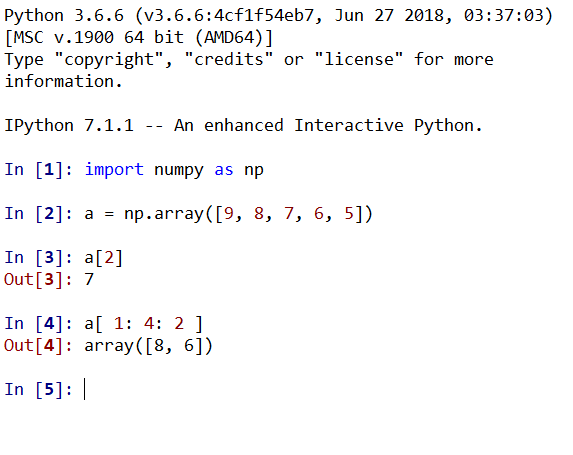多维数组的索引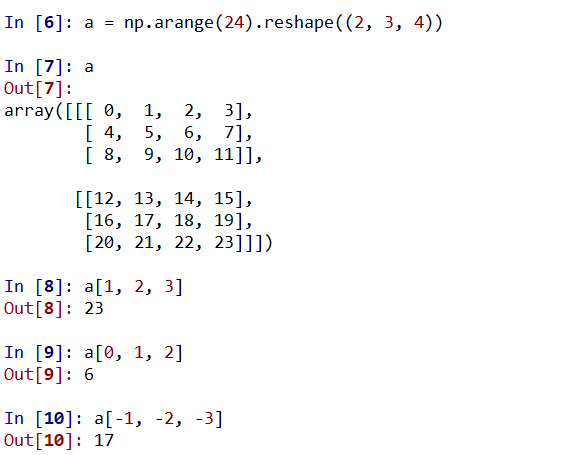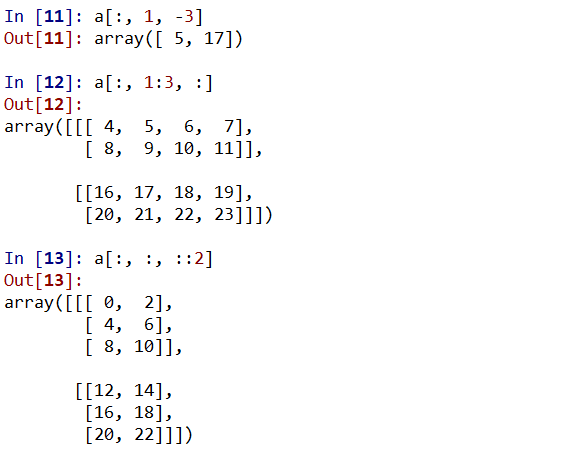4、ndarray数组的运算

数组与标量之间的运算：作用于数组每一个元素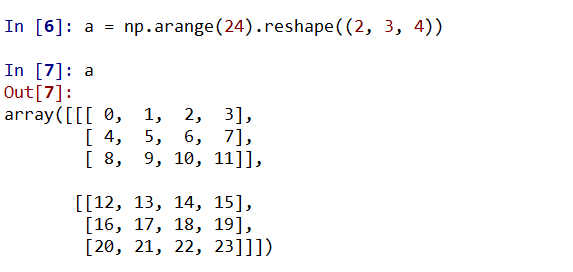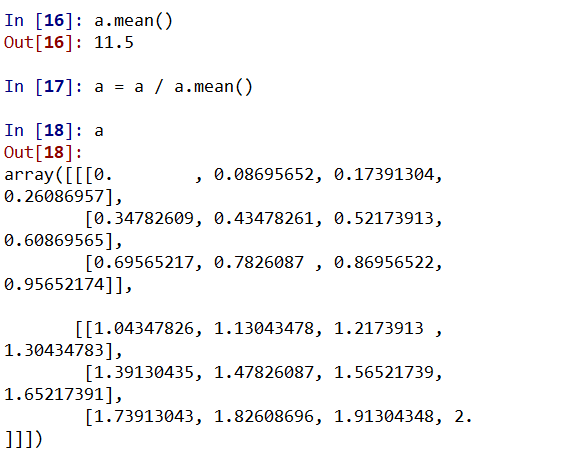Numpy一元函数对ndarray中的数据执行元素级运算的函数

np.abs(x) np.fabs(x) 计算数组各元素的绝对值
np.sqrt(x) 计算数组各元素的平方根
np.square(x) 计算数组各元素的平方
np.log(x) np.log10(x) np.log2(x) 计算数组各元素的自然对数、10底对数和2底对数
np.cell(x) np.floor(x) 计算数组各元素的celling值或floor值
np.rint(x) 计算数组各元素的四舍五入值
np.mod(x) 将数组各元素的小数和整数部分以两个独立数组形式返回
np.cos(x) np.cosh(x) np.sin(x) np.sinh(x) np.tan(x) np.tanh(x) 计算数组各元素的普通型和双曲型三角函数
np.exp(x) 计算数组各元素的指数值
np.sign(x) 计算数组各元素的符号值，1（+），0，-1（-）
Numpy二元函数

+ - * / ** 两个数组各元素进行对应运算
np.maximum(x,y) np.fmax() np.minimum(x,y) np.fmin() 元素级的最大值/最小值计算
np.mod(x,y) 元素级的模运算
np.copysign(x,y) 将数组y中各元素值的符号赋值给数组x对应元素
> < >= <= == != 算数比较，产生布尔型数组

二、Numpy数据存取与函数

1、数据的CSV文件存取

• fname：文件、字符串或生成器，可以是.gz或.bz2的压缩文件
• array：存入文件的数组
• fmt：写入文件的格式，例如：%d %.2f %.18e
• delimiter：分割字符串，默认是任何空格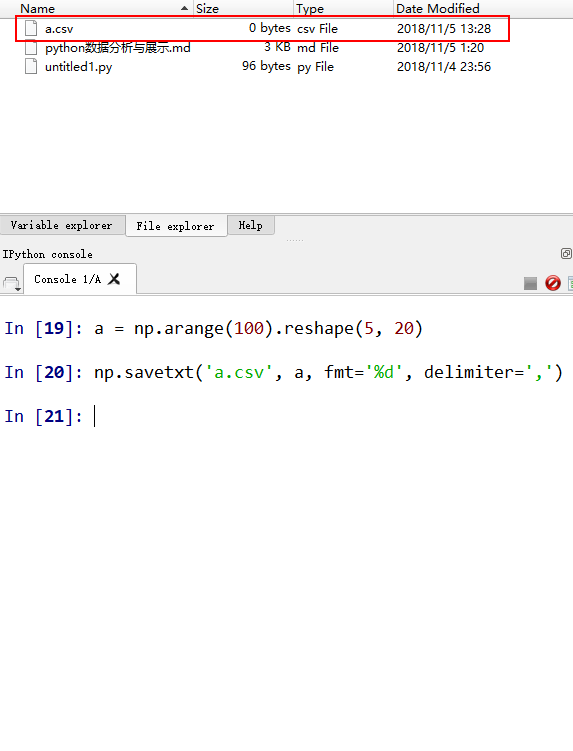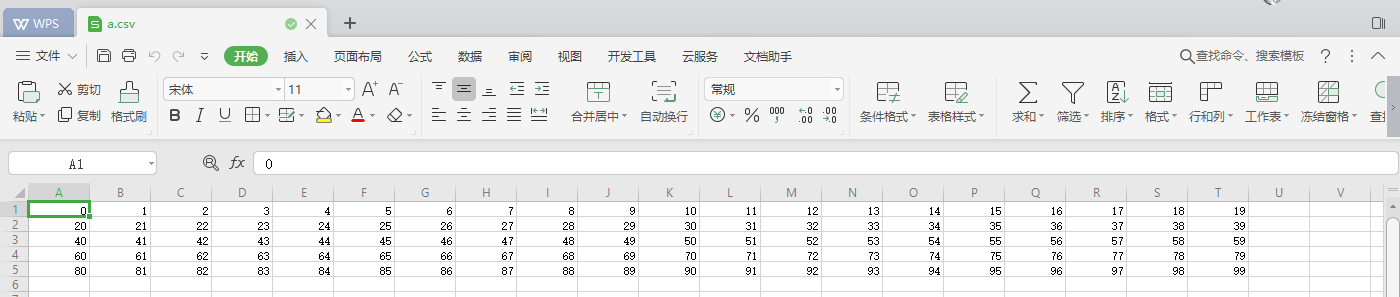• fname：文件、字符串或生成器，可以是.gz或.bz2的压缩文件
• dtype：数据类型，可选
• delimiter：分割字符串，默认是任何空格
• unpack：如果True，读入属性将分别写入不同变量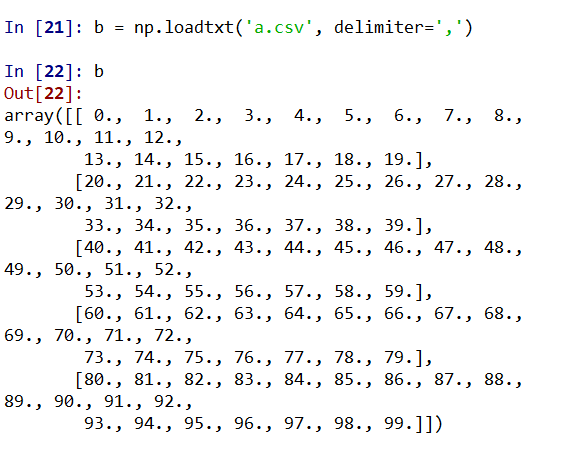CSV只能有效存储一维和二维数组

2、多维数据的存取

• fname：文件、字符串
• sep：数据分割字符串，如果是空串，写入文件为二进制
• format：写入数据的格式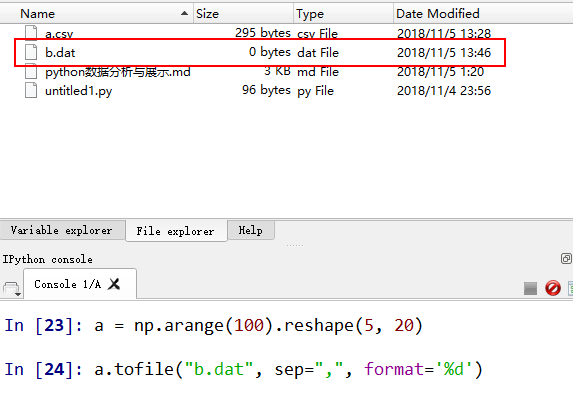• fname：文件、字符串
• dtype：读取的数据类型
• count：读入元素个数，-1表示读入整个文件
• sep：数据分割字符串，如果是空串，写入文件为二进制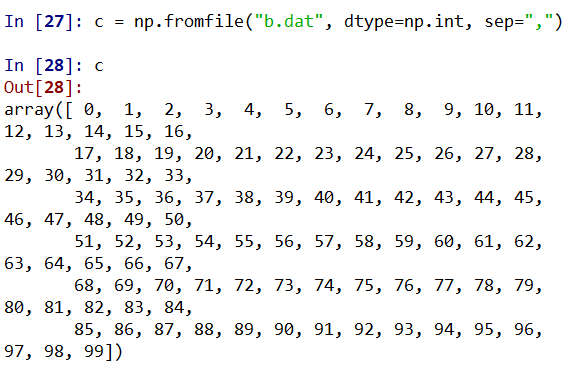a.tofile()np.fromfile()需要配合使用

Numpy的便捷文件存取

• fname：文件名，以.npy为扩展名，压缩扩展名为.npz
• array：数组变量
• fname：文件名，以.npy为扩展名，压缩扩展名为.npz

3、Numpy的随机数函数

np.random的随机数函数

rand(d0,d1,...,dn) 根据d0-dn创建随机数数组，浮点数，[0, 1)，均匀分布
randn(d0,d1,...,dn) 根据d0-dn创建随机数数组，标准正态分布
randint(low[,high,shape]) 根据shape创建随机数数组，范围是[low, high)
seed(s) 随机数种子，s是给定的种子值
shuffle(a) 根据数组a的第1轴进行随排列，改变数组a
permutation(a) 根据数组a的第1轴产生一个新的乱序数组，不改变数组a
choice(a[,size,replace,p]) 从一维数组a中以概率p抽取元素，形成size形状新数组，replace表示是否可以重用元素，默认为False
uniform(low,high,size) 产生具有均匀分布的数组，low起始值，high结束值，size形状
normal(loc,scale,size) 产生具有正态分布的数组，loc均值，scale标准差，size形状
poisson(lam,size) 产生具有泊松分布的数组，lam随机事件发生率，size形状

4、Numpy的统计函数

np.random的统计函数

sum(a,axis=None) 根据给定轴axis计算数组a相关元素之和，axis整数或元祖
mean(a,axis=None) 根据给定轴axis计算数组a相关元素的期望，axis整数或元祖
average(a,axis=None,weights=None) 根据给定轴axis计算数组a相关元素的加权平均值，weights是权值
std(a,axis=None) 根据给定轴axis计算数组a相关元素的标准差
var(a,axis=None) 根据给定轴axis计算数组a相关元素方差
min(a) max(a) 计算数组a中元素的最小值、最大值
argmin(a) argmax(a) 计算数组a中元素的最小值、最大值的降一维后下标
unravel_index(index,shape) 根据shape将一维下标index转换为多维下标
ptp(a) 计算数组a中元素最大值与最小值的查
median(a) 计算数组a中元素的中位数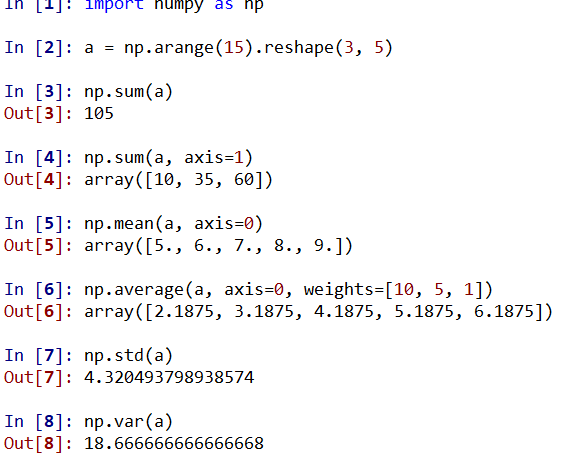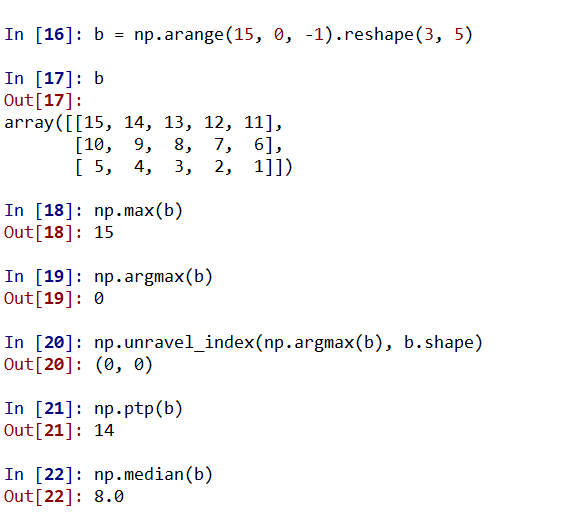实例：图像手绘效果数据分析——展示

一、Matplotlib库入门

1、Matplotlib库介绍

Matplotlib库效果：http://matplotlib.org/gallery.html

Matplotlib库的使用

Matplotlib库由各种可视化类构成，内部结构复杂，受MATLAB启发。

matplotlib.pyplot是绘制各种可视化图形的命令子库，相当于快捷方式。

pyplot的绘图区域

nrows横轴数量，ncols纵轴数量，plot_number绘图区域

2、pyplot的plot()函数

x X轴数据，列表或数组，可选
y Y轴数据，列表或数组
format_string 控制曲线的格式字符串，可选
**kwargs 第二组或更多组（x，y，format_string）format_string：有颜色字符、风格字符和标记字符组成

‘b’ 蓝色 ‘m’ 洋红色 magenta
‘g’ 绿色 ‘y’ 黄色
‘r’ 红色 ‘k’ 黑色
‘c’ 青绿色 cyan ‘w’ 白色
‘#008000’ RGB颜色 ‘0.8’ 灰度值字符串

‘-‘ 实线
’–‘ 破折线
’-.’ 点划线
‘:’ 虚线
‘’ 或 ‘ ’ 无线条

‘.’ 点标记 ’4‘ 右花三角标记
‘,’ 像素标记（极小点） ’s‘ 实心方形标记
’o‘ 实心圆标记 ’p‘ 实心五角标记
’v‘ 倒三角标记 ’*‘ 星形标记
’^’ 上三角标记 ’h‘ 竖六边形标记
‘>’ 右三角标记 ’H‘ 横六边形标记
’<‘ 左三角标记 ’+‘ 十字标记
’1‘ 下花三角标记 ’x‘ X标记
’2‘ 上花三角标记 ’D‘ 菱形标记
’3‘ 左花三角标记 ’d‘ 瘦菱形标记
’丨‘ 垂直线标记

**kwargs：第二组或更多组（x，y，format_string）

color 控制颜色
linestyle 线条风格
marker 标记风格
markerfacecolor 标记颜色

3、pyplot中文显示

第一种方法

font.family 用于显示 字体的名字
font.style 字体风格，正常'normal'或斜体 'italic'
font.size 字体大小 ，整数字号或者'large''x-small'

'SimHei' 中文黑体
'Kaiti' 中文楷体
'LiSu' 中文隶书
'FangSong' 中文仿宋
'YouYuan' 中文幼圆
'STSong' 华文宋体

3、pyplot的文本显示函数

plt.xlabel() 对X轴增加文本标签
plt.ylabel() 对Y轴增加文本标签
plt.title() 对图形整体增加文本标签
plt.text() 在任意位置增加文本标签
plt.annotate() 在图形中增加带箭头的注释二、Matplotlib基础函数

1、pyplot基础图表函数概述

plt.plot(x, y, fmt, ...) 绘制一个图标图
plt.boxplot(data, notch, position) 绘制一个箱型图
plt.bar(left, height, width, bottom) 绘制一个条形图
plt.barh(width, bottom, left, height) 绘制一个横向条形图
plt.polar(theta, r) 绘制极坐标图
plt.pie(datga, explode) 绘制饼图
plt.psd(x, NFFT=256, pad_to, Fs) 绘制功率谱密度图
plt.specgram(x, NFFT=256, pad_to, F) 绘制谱图
plt.cohere(x, y, NFFT=256, Fs) 绘制X-Y的相关性函数
plt.scatter(x, y) 绘制散点图，其中x和y长度相同
plt.step(x, y, where) 绘制步阶图
plt.hist(x, bins, normed) 绘制直方图
plt.contour(X, Y, Z, N) 绘制等值图
plt.vlines() 绘制垂直图
plt.stem(x, y, linefmt, markerfmt) 绘制柴火图
plt.plot_date() 绘制数据日期

2、pyplot饼图的绘制3、pyplot直方图4、pyplot极坐标图5、pyplot散点图三、实例：绘制引力波数据分析——概要

一、Pandas库入门

1、Pandas库介绍

Pandas官网

Pandas库的引用

Pandas是Python第三方库，提供高性能易用数据类型和分析工具

Pandas基于NumPy实现，常与NumPy和Matplotlib一同使用

Pandas库的理解

• 两个数据类型
• Seies
• DataFrame
• 基于上述数据类型的各种操作
• 基本操作
• 运算操作
• 特征类操作
• 关联类操作
NumPy Pandas

2、Pandas库的Series类型

Series类型• Python列表
• 标量值
• Python字典
• ndarray
• 其他函数
从标量值创建从字典类型创建从ndarray类型创建Serires类型的基本操作
• Series类型包括index和values两部分
• Series类型的操作类似ndarray类型
• 索引方法相同，采用[]
• NumPy中运算和操作可用于Series类型
• 可以通过自定义索引的列表进行切片
• 可以通过自动索引进行切片，如果存在自定义索引，则一同被切片
• Series类型的操作类似Python字典类型
• 通过自定义索引访问
• 保留字in操作
• 使用.get()方法Series类型对齐问题

Series + SeriesSeries类型在运算中会自动对齐不同索引的数据

Series类型的name属性

Series对象和索引都可以有一个名字，存储在属性.nameSeries类型的修改

Series对象可以随时修改并立即生效3、Pandas库的DataFrame类型

DataFrame类型

DataFrame类型由共用相同索引的一组列组成• DataFrame是一个表格型的数据类型，每列值类型可以不同
• DataFrame基友行索引，也有列索引
• DataFrame常用于表达二维数据，但也可以表达多维数据
• DataFrame类型可以由以下类型创建
• 二维ndarray对象
• 一维ndarray、列表、字典、元祖或Series构成的字典
• Series类型
• 其他DataFrame类型
从二维ndarray对象创建从一维ndarray对象字典创建从列表类型的字典创建4、Pandas库的数据类型操作

• 增加或重排：重新索引
• 删除：drop

重新索引

.reindex()能够改变或重排Series和DataFrame索引index, columns 新的行列自定义索引
fill_value 重新索引中，用于填充缺失位置的值
method 填充方法，ffill当前值向前填充，bfill向后填充
limit 最大填充量
copy 默认True，生成新的对象，False时，新旧相等不复制索引类型• Series和DataFrame的索引是Index类型
• Index对象是不可修改类型

.append(idx) 连接另一个Index对象，产生新的Index对象
.diff(idx) 计算差集，产生新的Index对象
.intersection(idx) 计算交集
.union(idx) 计算并集
.delete(loc) 删除loc位置处的元素
.insert(loc, e) 在loc位置增加一个元素e删除指定索引对象

.drop()能够删除Series和DataFrame指定行或列索引5、Pandas库的数据类型运算

算数运算法则

• 算术运算根据行列索引，补齐后运算，运算默认产生浮点数
• 补齐时缺项填充NaN
• 二维和一维、一维和零维间为广播运算
• 采用+-*/符号进行的二元运算产生新的对象.sub(d, **argws) 类型间减法运算，可选参数
.mul(d, **argws) 类型间乘法运算，可选参数
.div(d, **argws) 类型间除法运算，可选参数fill_value参数代替NaN，替代后参与运算比较运算

• 比较运算只能比较相同索引的元素，不进行补齐
• 二维和一维、一维和零维间为广播运算
• 采用> < >= <= == !=等符号进行的二元运算产生布尔对象二、Pandas数据特征分析

1、数据的排序

• .sort_index()方法在指定轴上根据索引进行排序，默认升序
• .sort_index(axis=0, ascending=True)• .sort_values()方法在指定轴上根据数值进行排序，默认升序
• Series.sort_values(axis=0, ascending=True)
• DataFrame.sort_values(by, axis=0, ascending=True)
• by：axis轴上的某个索引或索引列表• NaN同意放到排序末尾

2、数据的基本统计分析

适用于Series和DataFrame类型

.sum() 计算数据的总和，按0轴就算，下同
.count() 非NaN值的数量
.mean() .median() 计算数据的算数平均值、算数中位数
.var() .std() 计算数据的方差、标准差
.min() .max() 计算数据的最小值、最大值
describe() 针对0轴（各列）的统计汇总

适用于Series类型

.argmin() .argmax() 计算数据最大值、最小值所在位置的索引位置（自动索引）
.idxmin() .idxmax() 计算数据最大值、最小值所在位置的索引（自定义索引）3、数据的累计统计分析

.cumsum() 前n个数和
.cumprod() 前n个数积
.cummax() 前n个数最大值
.cummin() 前n个数最小值

滚动计算（窗口计算）函数

.rolling(w).sum() 依次计算相邻w个元素的和
.rolling(w).mean() 依次计算相邻w个元素的算数平均值
.rolling(w).var() 依次计算相邻w个元素的方差
.rolling(w).std() 依次计算相邻w个元素的标准差
.rolling(w).min() .rolling(w).max() 依次计算相邻w个元素的最大值和最小值

4、分析数据相关性相关分析函数

.cov() 计算协方差矩阵
.corr() 计算相关系数矩阵，Pearson、Spearman、Kendall等系数